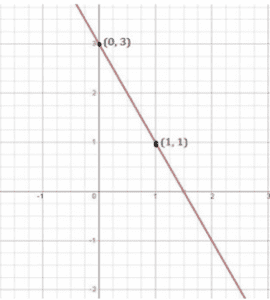Newbie

# Draw the graph of each of the following linear equations in two variables:(iv) 3 = 2x+y . Q.1(4)

• 0

Find the simplest solution of ncert class 9th solution of linear equations in two variables of exercise 4.3 question number 1(4). Sir please help me to solve this question in a easiest and best way. Find the best solution of this question. Draw the graph of each of the following linear equations in two variables:(iv) 3 = 2x+y .

Share

1. Solution:

To draw a graph of linear equations in two variables, let us find out the points to plot.

To find out the points, we have to find the values which x and y can have, satisfying the equation.

Here,

3 = 2x+y

Substituting the values for x,

When x = 0,

3 = 2x+y

3 = 2×0+y

3 = 0+y

y = 3

When x = 1,

3= 2x+y

3 = 2×1+y

3 = 2+y

y = 3–2

y = 1

 x y 0 3 1 1

The points to be plotted are (0, 3) and (1, 1)• 0